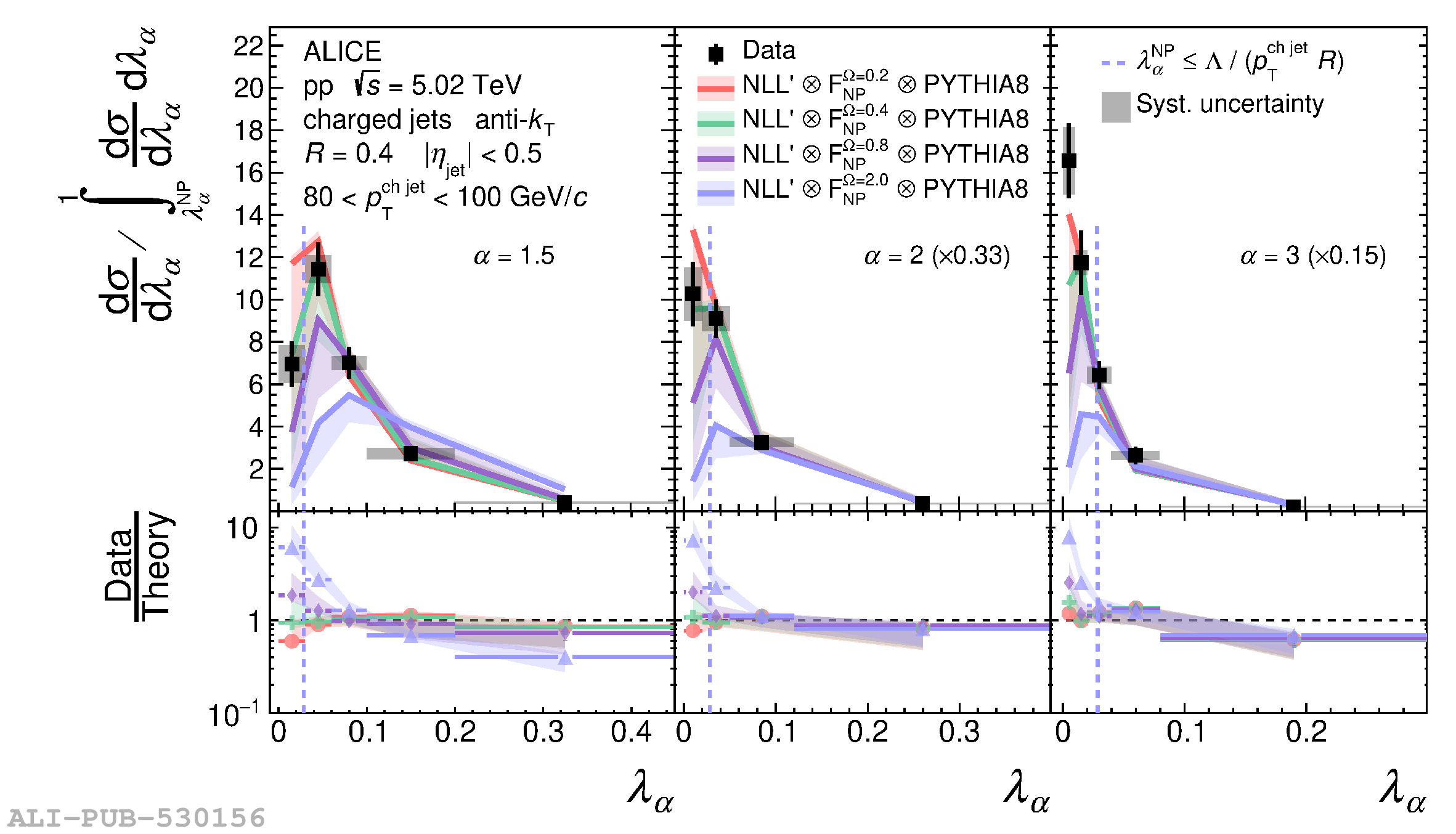# Figure A.4

 Comparison of ungroomed jet angularities \ang{} in \pp{}collisions for $R=0.2$ (top) and $R=0.4$ (bottom) to analyticalNLL$^\prime$ predictions using $F(k)$ convolution in therange $80 < \pTchjet{} < 100$ \GeVc{}. The distributions arenormalized such that the integral of the perturbative region definedby $\ang > \angNP{}$ (to the right of the dashed vertical line)is unity. Divided bins are placed into the left (NP) region.